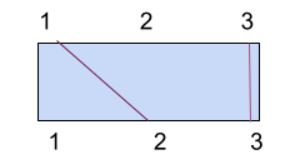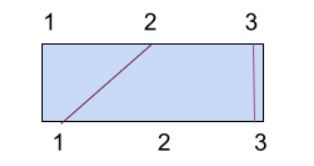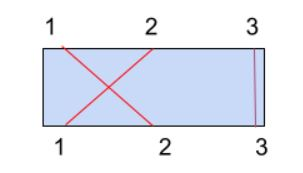1

# Connecting Ropes

Difficulty: HARDContributed By
Ashish Kumar
Avg. time to solve
20 min
Success Rate
80%

Problem Statement

#### Given an integer ‘N’ and arrays ‘A’ and ‘B’ representing the coordinates of houses. Find the maximum number of pairs of houses Ninja can connect.

##### Example :
``````N = 3
A = [ 1, 2, 3 ]
B = [ 2, 1, 3 ]

Explanation :

One of the possible connections can be (1,2) and (3,3).
````````````Another possible connection is (2,1) and (3,3).
````````````Ninja cannot connect all 3 pairs (1,2) , (2,1) and (3,3) as the first 2 pairs cross each other.
````````````So, the maximum connection is 2.
``````
##### Input Format :
``````The first line contains an integer 'T' which denotes the number of test cases to be run. Then the test cases follow.

The first line of each test case contains an integer ‘N’.

The next line contains ‘N’ integers representing the elements of array ‘A’ which denotes the coordinates of the northern houses.

The next line contains ‘N’ integers representing the elements of array ‘B’ which denotes the coordinates of the southern  houses.
``````
##### Output format :
``````For each test case, output an integer denoting the maximum connections of houses possible.

Print the output of each test case in a new line.
``````
##### Note :
``````You don’t need to print anything. It has already been taken care of. Just implement the given function.
``````
##### Constraints :
``````1 <= T <= 5
1 <= N <= 10^5
1 <= A[i] <= 10^5

Time Limit: 1 sec
``````
##### Sample Input 1 :
``````2
2
1 2
1 2
3
1 2 3
3 2 1
``````
##### Sample Output 1 :
``````2
1
``````
##### Explanation Of Sample Input 1 :
``````For test case 1 we have,

Both the pairs (1,1) and (2,2) can be connected without any ropes crossing each other.

So, we output 2.

For test case 2 we have,

Any two pairs of houses will cross with each other.

So, we can only connect 1 of the 3 pairs of houses.

So, we output 1.
``````
##### Sample Input 2 :
``````2
4
5 4 7 2
5 3 4 2
3
6 4 3
3 7 5
``````
##### Sample Output 2 :
``````3
2
``````Console